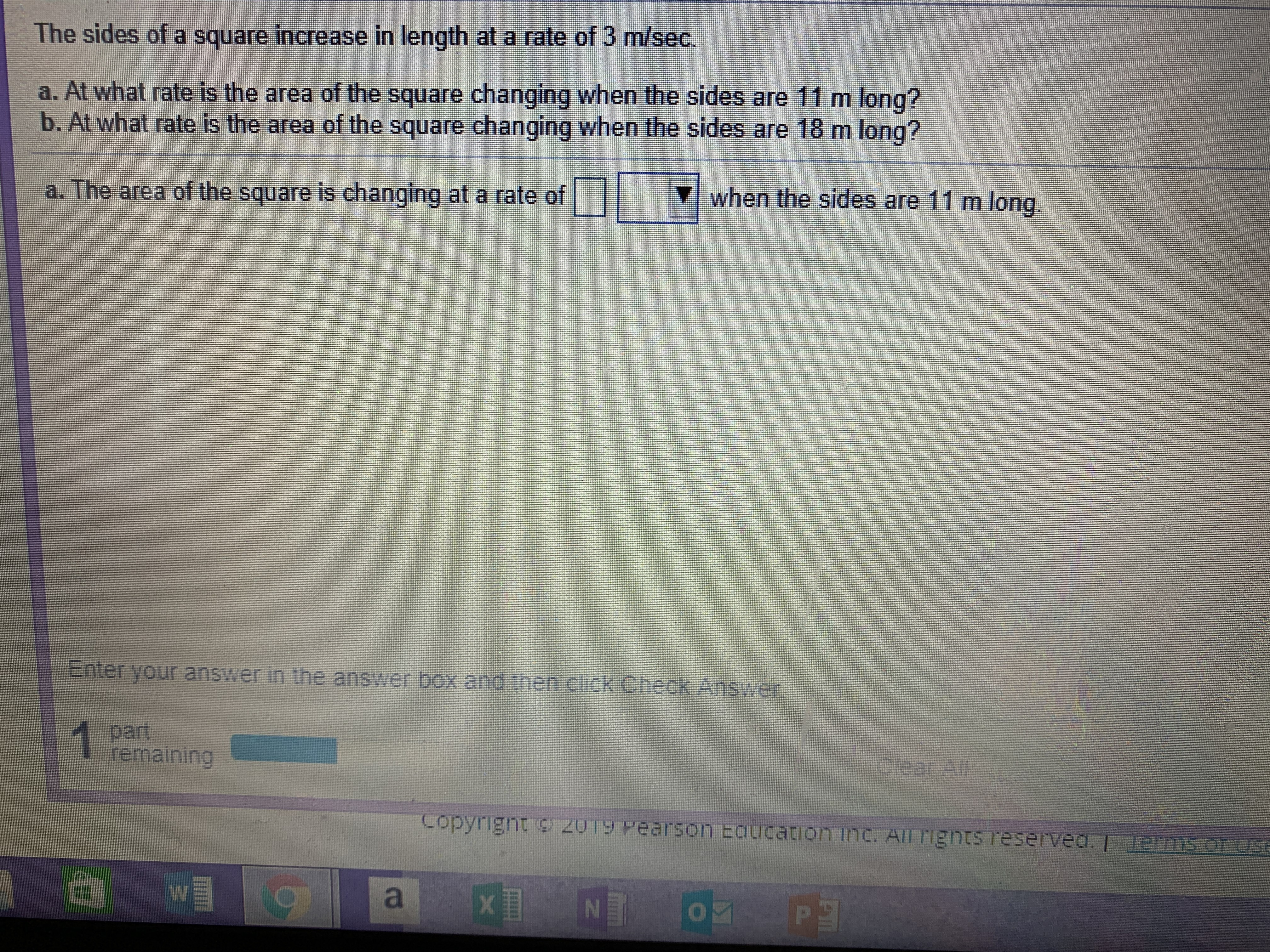# The sides of a square increase in length at a rate of 3 m/sec.a. At what rate is the area of the square changing when the sides are 11 m long?b. At what rate is the area of the square changing when the sides are 18 m long?a. The area of the square is changing at a rate ofY when the sides are 11 m longEnter your answer in the answer box and then click Check AnswerremainingCopyrignt2u1g wearson Eaucation inc. ATFrignts reserved. I

Question
29 viewshelp_outlineImage TranscriptioncloseThe sides of a square increase in length at a rate of 3 m/sec. a. At what rate is the area of the square changing when the sides are 11 m long? b. At what rate is the area of the square changing when the sides are 18 m long? a. The area of the square is changing at a rate of Y when the sides are 11 m long Enter your answer in the answer box and then click Check Answer remaining Copyrignt 2u1g wearson Eaucation inc. ATFrignts reserved. I fullscreen
check_circle

Step 1

Let  l be the length of the square.

The area of the square is A=l^2 .

The rate of change in lengt...

### Want to see the full answer?

See Solution

#### Want to see this answer and more?

Solutions are written by subject experts who are available 24/7. Questions are typically answered within 1 hour.*

See Solution
*Response times may vary by subject and question.
Tagged in

### Derivative